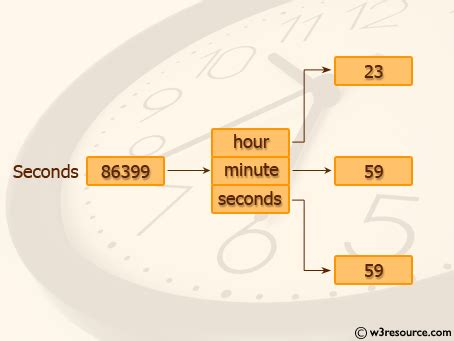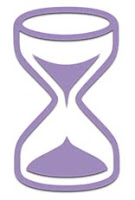Write a program to convert age in seconds to hours and minutes in python.for this we have to use a module of python that is called datetime .after that we need some details from the user like month,day and year.

Now before we start our program we have to understand the logic behind the program.

To know the birth date,current date between time we have to do:-import datetime
current_date = datetime.datetime.now()

del = input('Enter Date of birth (mm/dd/yyyy)')
delDate = datetime.datetime.strptime(del,'%m%d&y')
print(deldate)
days_left = deldate - current_Date
print(days_left)

This will give us the left days between the birth and current date.

Python provides datetime module to deal with all datetime related issues in python. Using datetime we can find the age by subtracting birth year from current year. Along with this, we need to focus on the birth month and birthday. For this, we check if current month and date are less than birth month and date. If yes subtract 1 from age, otherwise 0.

Now we have to calculate the years,months,days,hours,minutes and seconds of the age .for this we have to do some mathematical calculations like:-

year = ((days_left.total_seconds())/(365.242*24*3600))
yrs_int = int(year)

month = (year-yrs_int)*12
month_int = int(month)

days = (month_int)*(365.242/12)
days_int=int(days)

hours = (days-days_int)*24
hours_int = int(hours)

min = (hours-hours_int)*60
min_int = int(min)

seconds = (min-min_int)*60
seconds_int = int(seconds)

Now the whole program is:-

import datetime
current_date = datetime.datetime.now()

del = input('Enter Date of birth (mm/dd/yyyy)')
delDate = datetime.datetime.strptime(del,'%m%d&y')
print(deldate)
days_left = deldate - current_Date
print(days_left)

year = ((days_left.total_seconds())/(365.242*24*3600))
yrs_int = int(year)

month = (year-yrs_int)*12
month_int = int(month)

days = (month_int)*(365.242/12)
days_int=int(days)

hours = (days-days_int)*24
hours_int = int(hours)

min = (hours-hours_int)*60
min_int = int(min)

seconds = (min-min_int)*60
seconds_int = int(seconds)

print' You are {0:d} years, {1:d} months, {2:d} days, {3:d} hours, {4:d} minutes, {5:d} seconds old.format(yrs_int,month_int,days_int,hours_int,min_int,seconds_int))